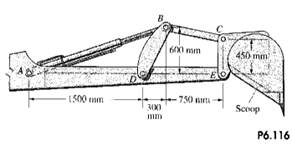### Create an Account

Home / Questions / The angular velocity of the scoop is 10rads clockwise Determine the rate at which the hydr...

# The angular velocity of the scoop is 10rads clockwise Determine the rate at which the hydraulic actuator AB is extending 2 The angular acceleration of the scoop in Problem 6.116 is zero

The angular velocity of the scoop is 1.0rad/s clockwise. Determine the rate at which the hydraulic actuator AB is extending.2. The angular acceleration of the scoop in Problem 6.116 is zero.

determine the rate of change of the rate at which the hydraulic actuator AB is extending

Problem 6.116

The angular velocity of the scoop is 1.0rad/s clockwise. Determine the rate at which the hydraulic actuator AB is extending.Subscribe To Get Solution